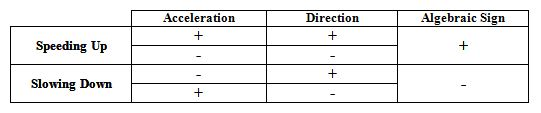$$\require{cancel}$$

# 01. Algebraic Signs

$$\newcommand{\vecs}{\overset { \rightharpoonup} {\mathbf{#1}} }$$ $$\newcommand{\vecd}{\overset{-\!-\!\rightharpoonup}{\vphantom{a}\smash {#1}}}$$$$\newcommand{\id}{\mathrm{id}}$$ $$\newcommand{\Span}{\mathrm{span}}$$ $$\newcommand{\kernel}{\mathrm{null}\,}$$ $$\newcommand{\range}{\mathrm{range}\,}$$ $$\newcommand{\RealPart}{\mathrm{Re}}$$ $$\newcommand{\ImaginaryPart}{\mathrm{Im}}$$ $$\newcommand{\Argument}{\mathrm{Arg}}$$ $$\newcommand{\norm}{\| #1 \|}$$ $$\newcommand{\inner}{\langle #1, #2 \rangle}$$ $$\newcommand{\Span}{\mathrm{span}}$$ $$\newcommand{\id}{\mathrm{id}}$$ $$\newcommand{\Span}{\mathrm{span}}$$ $$\newcommand{\kernel}{\mathrm{null}\,}$$ $$\newcommand{\range}{\mathrm{range}\,}$$ $$\newcommand{\RealPart}{\mathrm{Re}}$$ $$\newcommand{\ImaginaryPart}{\mathrm{Im}}$$ $$\newcommand{\Argument}{\mathrm{Arg}}$$ $$\newcommand{\norm}{\| #1 \|}$$ $$\newcommand{\inner}{\langle #1, #2 \rangle}$$ $$\newcommand{\Span}{\mathrm{span}}$$

Confusion about the meaning of algebraic signs is common among beginning physics students. The best way to clarify this confusion is to remember that algebraic signs are simply a mathematical way to describe direction. Instead of saying up and down, or east and west, physicists construct coordinate systems and translate the words east and west into the symbols ‘+’ and ‘-’, or even ‘-’ and ‘+’ if we choose a different coordinate system. The key to the translation is the coordinate system. A coordinate system is very similar to the English-French dictionary you might take with you on your first trip to France. When you see a ‘-’, use your coordinate system to translate it into a verbal description of direction.

Do not fall into the common habit of translating a ‘-’ into the word “decreasing”. A negative acceleration, for example, does NOT imply that the object is slowing down. It implies an acceleration that points in the negative direction. It is impossible to determine whether an object is speeding up or slowing down by looking at the sign of the acceleration! Conversely, the word “deceleration”, which does mean that an object is slowing down, does not give any information regarding the sign of the acceleration. I can decelerate in the positive direction as easily as I can decelerate in the negative direction.

A guide to determining the correct algebraic sign:01. Algebraic Signs is shared under a CC BY-NC-SA license and was authored, remixed, and/or curated by Paul D'Alessandris.